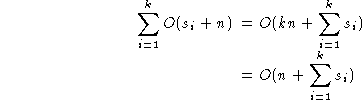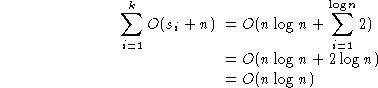Data Structures and Algorithms 7.5 Radix Sorting

The bin sorting approach can be generalised in a technique that is known as radix sorting.

#### An example

Assume that we have n integers in the range (0,n2) to be sorted. (For a bin sort, m = n2, and we would have an O(n+m) = O(n2) algorithm.) Sort them in two phases:
1. Using n bins, place ai into bin ai mod n,
2. Repeat the process using n bins, placing ai into bin floor(ai/n), being careful to append to the end of each bin.
This results in a sorted list.

As an example, consider the list of integers:

```36 9 0 25 1 49 64 16 81 4
```
n is 10 and the numbers all lie in (0,99). After the first phase, we will have:

Bin01 23 45 67 89
Content 0 1
81
-- 64
4
25 36
16
- - 9
49

Note that in this phase, we placed each item in a bin indexed by the least significant decimal digit.

Repeating the process, will produce:

Bin01 23 45 67 89
Content 0
1
4
9
16 25 36 49 - 64 - 81 -

In this second phase, we used the leading decimal digit to allocate items to bins, being careful to add each item to the end of the bin.

We can apply this process to numbers of any size expressed to any suitable base or radix.

We can further observe that it's not necessary to use the same radix in each phase, suppose that the sorting key is a sequence of fields, each with bounded ranges, eg the key is a date using the structure:
```typedef struct t_date {
int day;
int month;
int year;
} date;
```
If the ranges for day and month are limited in the obvious way, and the range for year is suitably constrained, eg 1900 < year <= 2000, then we can apply the same procedure except that we'll employ a different number of bins in each phase. In all cases, we'll sort first using the least significant "digit" (where "digit" here means a field with a limited range), then using the next significant "digit", placing each item after all the items already in the bin, and so on.

Assume that the key of the item to be sorted has k fields, fi|i=0..k-1, and that each fi has si discrete values, then a generalised radix sort procedure can be written:

 ```radixsort( A, n ) { for(i=0;ifi] }``` O(n) ``` for(j=0;j
 TotalNow if, for example, the keys are integers in (0,bk-1), for some constant k, then the keys can be viewed as k-digit base-b integers.
Thus, si = b for all i and the time complexity becomes O(n+kb) or O(n). This result depends on k being constant.

If k is allowed to increase with n, then we have a different picture. For example, it takes log2n binary digits to represent an integer <n. If the key length were allowed to increase with n, so that k = logn, then we would have:.

Another way of looking at this is to note that if the range of the key is restricted to (0,bk-1), then we will be able to use the radixsort approach effectively if we allow duplicate keys when n>bk. However, if we need to have unique keys, then k must increase to at least logbn. Thus, as n increases, we need to have logn phases, each taking O(n) time, and the radix sort is the same as quick sort!

### Sample code

This sample code sorts arrays of integers on various radices: the number of bits used for each radix can be set with the call to SetRadices. The Bins class is used in each phase to collect the items as they are sorted. ConsBins is called to set up a set of bins: each bin must be large enough to accommodate the whole array, so RadixSort can be very expensive in its memory usage!